# RS Aggarwal Solutions for Class 9 Maths Chapter 5 - Congruence of Triangles Ex 5B (5.2)

## RS Aggarwal Solutions for Class 9 Maths Chapter 5 – Congruence of Triangles Ex 5B (5.2) Solutions Free PDF

Question 1:

In the given figure, if x = y and AB = CB, then prove that AE = CD.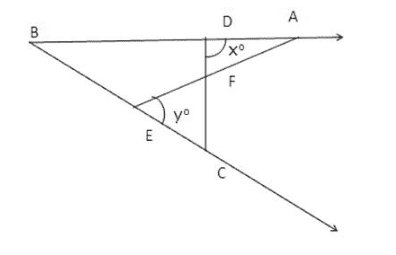Solution:

Given: AB = BC

and, xo = yo

To prove: AE = CD

Proof: In $\Delta$ABE, we have,

Exterior $\angle$AEB = $\angle$EBA + $\angle$BAE

$\Rightarrow$ yo = $\angle$EBA + $\angle$BAE

Again, in $\Delta$BCD, we have

xo = $\angle$CBA + $\angle$BCD

Since, xo = yo [Given]

So, $\angle$EBA + $\angle$BAE = $\angle$CBA + $\angle$BCD

$\Rightarrow$ $\angle$BAE = $\angle$BCD

Thus in $\Delta$BCD and $\Delta$BAE, we have

$\angle$B = $\angle$B [Common]

BC = AB [Given]

and, \)$\angle$BAE = $\angle$BCD [Proved above]

Thus by Angle-Side-Angle criterion of congruence, we have

$\Delta$BCD $\cong$ $\Delta$BAE

The corresponding parts of the congruent triangles are equal.

So, CD = AE [Proved]

Question 2:

ABC is a triangle in which AB = AC. If the bisectors of $\angle$B and $\angle$C meet AC and AB in D and E respectively, prove that BD = CE.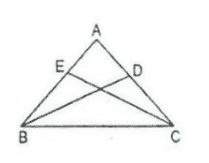Solution:

Given: A $\Delta$ABC in which AB = AC and BD and CE are the bisectors of $\angle$B and $\angle$C respectively.

To prove: BD = CE

Proof: In $\Delta$ABC and $\Delta$ACE

$\angle$ABD = ½ $\angle$B

and, $\angle$ACE = ½ $\angle$C

But $\angle$B = $\angle$C as AB = AC [In isosceles triangle, base angles are equal]

$\Rightarrow$ $\angle$ABD = $\angle$ACE

AB = AC [Given]

$\angle$A = $\angle$A [Common]

Thus by Angle-Side-Angle criterion of congruence, we have

$\Delta$ABD $\cong$ $\Delta$ACE

The corresponding parts of the congruent triangles are equal.

BD = CE [c.p.c.t]

Question 3:

In the adjoining figure, AD is a median of $\Delta$ABC. If BL and CM are drawn perpendiculars on AD and AD produced, prove that BL = CM.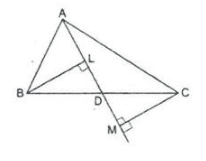Solution:

Given: A triangle in which D is the midpoint of BC and BL $\perp$ AD and CM $\perp$ AD

To prove: BL = CM

Proof: In $\Delta$BLD and $\Delta$CMD

$\angle$BLD = $\angle$CMD = 90o [Given]

$\angle$BDL = $\angle$MDC [Vertically opposite angles]

BD = DC [Given]

Thus by Angle-Angle-Side criterion of congruence, we have

$\Delta$BLD $\cong$ $\Delta$CMD

The corresponding parts of the congruent triangles are equal

So, BL = CM [c.p.c.t]

Question 4:

In $\Delta$ABC, D is the mid-point of BC. If DL $\perp$ AB and DM $\perp$ AC such that DL = DM, prove that AB = AC.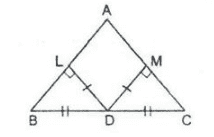Solution:

Given: In a $\Delta$ABC, D is the midpoint of BC and DL $\perp$ AB and DM $\perp$ AC. Also, DL = DM

To prove: AB = AC

Proof: In right angled triangle $\Delta$BLD and $\Delta$CMD

$\angle$BLD = $\angle$CMD = 90o

Hypt. BD = Hypt. CD [Given]

DL = DM [Given]

Thus, by Right Angle-Hypotenuse_side criterion of congruence, we have

$\Delta$BLD $\cong$ $\Delta$CMD

The corresponding parts of the congruent triangles are equal.

Therefore, $\angle$ABD = $\angle$ACD [c.p.c.t]

In $\Delta$ABC, we have

$\angle$ABD = $\angle$ACD

$\Rightarrow$ AB = AC [Since opposite to equal angles are equal]

Question 5:

In $\Delta$ABC, AB = AC and the bisectors of $\angle$B and $\angle$C meet at a point O. Prove that BO = CO and the ray AO is the bisector of $\angle$A.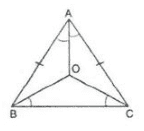Solution:

Given: A $\Delta$ABC in which AB = AC, BO and CO are bisectors of $\angle$B and $\angle$C

To prove: In $\Delta$BOC, we have,

$\angle$OBC = ½ $\angle$B

and, $\angle$OCB = ½ $\angle$C

But, $\angle$B = $\angle$C [therefore AB = AC (given)]

So, $\angle$OBC = $\angle$OCB

Since base angles are equal, sides are equal

$\Rightarrow$ OB = OC ——- (1)

Since OB and OC are the bisectors of angles,

$\angle$B and $\angle$C respectively, we have

$\angle$ABO = ½ $\angle$B

$\angle$ACO = ½ $\angle$C

$\Rightarrow$ $\angle$ABO = $\angle$ACO  ——— (2)

Now, in $\Delta$ABO and $\Delta$ACO

AB = AC [Given]

$\angle$ABO = $\angle$ACO [from (2)]

BO = OC [from(1)]

Thus, by Side-Angle-Side criterion of congruence, we have

$\Delta$ABO $\cong$ $\Delta$ACO

The corresponding parts of the congruent triangles are equal

Therefore, $\angle$BAO = $\angle$CAO [by c.p.c.t]

i.e. , AO bisects $\angle$A.

Question 6:

In the given figure, PQR is an equilateral triangle and QRST is a square. Prove that

(i) PT = PS

(ii) $\angle$PSR = 15o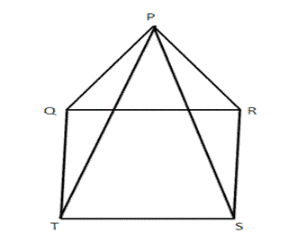Solution:

Given: PQR is an equilateral triangle and QRST is a square.

To prove: PT = PS

and $\angle$PSR = 15o

Proof: Since $\Delta$ is an equilateral triangle,

$\angle$ = 60o and $\angle$PRQ = 60o

Since QRTS is a square,

$\angle$RQT = 90o and $\angle$QRS = 90o

In $\Delta$PQT

$\angle$PQT = $\angle$PQR + $\angle$RQT

= 60o + 90o = 150o

In $\Delta$PRS

$\angle$PRS = $\angle$PRQ + $\angle$QRS

=60o + 90o = 150o ——- (1)

$\Rightarrow$ $\angle$PQT = $\angle$PRS ——— (2)

Thus, in $\Delta$PQT and $\Delta$PRS

PQ = RS [sides of equilateral triangle PQR]

$\angle$PQT = $\angle$PRS ——– (2)

QT = RS [sides of square QRST]

Thus, by Side-Angle-Side criterion of congruence, we have

therefore,  $\Delta$PQT $\cong$ $\Delta$PRS

The corresponding parts of the congruent triangles are equal.

Therefore, PT = PS [c.p.c.t]

Now in $\Delta$PRS, we have

PR = RS

$\Rightarrow$ $\angle$ RPS = $\angle$PSR

But $\angle$PRS = 150o [from(1)]

So, by angle sum property in $\Delta$PRS

$\angle$PRS + $\angle$SPR + $\angle$PSR = 180o

$\Rightarrow$ 150o + $\angle$PSR + $\angle$PSR = 180o

$\Rightarrow$ 2$\angle$PSR = 180o – 150o

$\Rightarrow$ $\angle$PSR = 30o/2 = 15o

Question 7:

In the given figure, ABC is a triangle, right angled at B. If BCDE is a square on side BC and ACFG is a square on AC, prove that AD = BF.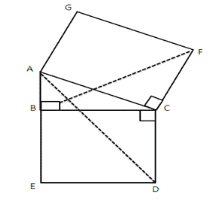Solution:

Given: ABC is a triangle right angled at B. ACFG is a square and BCDE is a square.

To prove: AD = EF

Proof: Since BCDE is a square,

$\angle$BCD = 90o ——- (1)

In $\Delta$ACD,

$\angle$ACD = $\angle$ACB + $\angle$BCD

= $\angle$ACB + 90o ——- (2)

In $\Delta$BCF,

$\angle$BCF = $\angle$BCA + $\angle$ACF

Since ACFG is a square,

$\angle$ACF = 90o

Thus, we have

$\angle$BCF = $\angle$BCA + 90o ——- (3)

From (2) and (3), we have

$\angle$ACD = $\angle$BCF ——— (4)

Thus in $\Delta$ACD and $\Delta$BCF, we have

AC = CF [sides of a square]

$\angle$ACD = $\angle$BCF [from (4)]

CD = BC [sides of a square]

Thus, by Side-Angle-Side criterion of congruence, we have

Therefore, $\Delta$ACD $\cong$ $\Delta$BCF

The corresponding parts of congruent triangles are equal.

So, AD = BF (c.p.c.t)

Question 8:

Prove that the median from the vertex of an isosceles triangle is the bisector of the vertical angle.

Solution:

Given: ABC is a isosceles triangle in which AB = AC and AD is the median through A.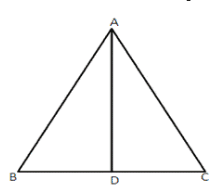To prove: $\angle$BAD = $\angle$DAC

Proof: In $\Delta$ABD and $\Delta$ADC

AB = AC [Given]

BD = DC [Given]

Thus by Side-Side-Side criterion of congruence, we have

$\Delta$ABD $\cong$ $\Delta$ADC

The corresponding parts of the congruent triangles are equal.

Therefore, $\angle$BAD = $\angle$DAC (Proved)

Question 9:

In the given figure, ABCD is quadrilateral in which AB || DC and P is the mid-point of BC. On producing, AO and DC meet at Q. Prove that

(i) AB = CQ

(ii) DQ = DC + AB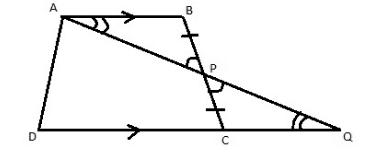Solution:

Given ABCD is a quadrilateral in which AB || DC

To prove:

i) AB = CQ

ii) DQ = DC + AB

Proof: In $\Delta$ABP and $\Delta$PCQ we have

$\angle$PAB = $\angle$PQC [alternate angles]

$\angle$APB = $\angle$CPQ [vertically opposite angles]

BP = PC [Given]

Thus by Angle-Angle-Side criterion of congruence, we have

$\Delta$ABP $\cong$ $\Delta$PCQ

The corresponding parts of the congruent triangles are equal

Therefore, AB = CQ ——– (1)

Now, DQ = DC + CQ

= DC + AB [from (1)]

Question 10:

In the given figure, OA = OB and OP = OQ. Prove that

(i) PX = QX

(ii) AX = BX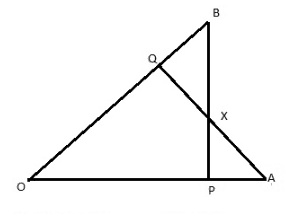Solution:

Given: OA = OB and OP = OQ

To prove:

(i) PX = QX

(ii) AX = BX

Proof: In $\Delta$OAQ and $\Delta$OPB, we have,

OA = OB [Given]

$\angle$O = $\angle$O [Common]

OQ = OP [Given]

Thus by Side-Angle-Side criterion of congruence, we have

$\Delta$OAQ $\cong$ $\Delta$OPB

The corresponding parts of the congruent triangles are equal.

Therefore, $\angle$OBP = $\angle$OAQ ——— (1)

Thus, in $\Delta$BXQ and $\Delta$PXA, we have

BQ = OB – OQ

and, PA = OA – OP

But, OP = OQ

and OA =OB [Given]

Therefore, we have, BQ = PA ———- (2)

Now consider triangles $\Delta$BXQ and $\Delta$PXA.

$\angle$BXQ = $\angle$PXA [vertically opposite angles]

$\angle$OBP = $\angle$OAQ [from (1)]

BQ = PA [from (2)]

Thus by Angle-Angle-Side criterion of congruence, we have,

Therefore, $\Delta$BXQ $\cong$ $\Delta$PPXA

PX = QX [c.p.c.t]

AX = BX [c.p.c.t]